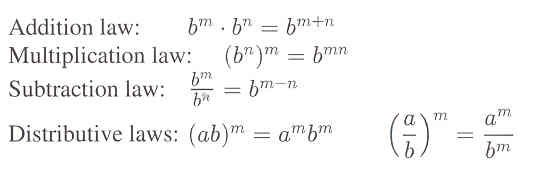# Section 1.3: Scientific Notation and Estimation

## 1.3 Outline

1. Exponential notation (see below)
1. base
2. exponent
3. power or exponential
4. extended order of operations
2. Scientific notation (see below)
3. Calculators
1. arithmetic logic
2. algebraic logic
3. RPN logic
4. floating-point form
4. Estimation
5. Laws of exponents (see below)
2. multiplication law
3. subtractive law
4. distributive law
6. Comprehending large numbers
1. million
2. billion
3. trillion

## 1.3 Essential Ideas

Exponents and Laws of Logarithms
Exponents play a central role in mathematical notation. Especially pay attention to the definition notation: For any number b and any counting number n:Closely related to exponential notation, there are five laws of exponents:
Let a and b be any nonzero real numbers, and let m and n be any integers. Then,Scientific Notation
The scientific notation for a nonzero number is that number written as a power of 10 times another number x, such that x is between 1 and 10, including 1.